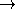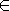# OEF gradient --- Introduction ---

This module actually contains 5 exercises on the gradient of 2 variables fonctions.

Let [,]be a parametric curve of equations

for[,].

and let be a function from to such that

for[,].

We give the following values :

What is the value of ? Can the gradient of at point be non zero ? Give the possible values of the slope of the gradient of at point in the case when it is non zero. If there is several cases, write all values (separate them with comma). Indeed the gradient of at point is zero. We assume that is in . Compute

and decide if admits a local extremum at

Here are some equidistant level curves of the function defined by

.

Compute the direction at the point at the level curve passing through point (give out the slope to close, if it is finite and inf if it is infinite).
xrange -, + yrange -, + parallel -,-,+,-,0,/10,20,grey parallel -,-,-,+,/10,0,20,grey arrow 0,0, 0,,10,black arrow 0,0, , 0 ,10,black vline 0,0, black hline 0,0, black levelcurve magenta, , levelcurve blue, , disk ,, 5,blue text black, ,, giant, A

Here are some level curves of defined with

drawn with a step and two points et are given and drawn. Is the gradient of of larger norm at point or at point ?
xrange -, yrange -, parallel -,-,,-, 0,0.5, *20, grey parallel -,-,-,, 0.5,0, *20, grey arrow -,0,,0,10,black arrow 0,-,0,,10,black levelcurve magenta,, disk ,, 5, blue disk ,, 5, blue text black, ,medium, text black, ,medium,

We call isotherms the level curves of the functionand adiabatics the level curves of the function.

• On what is the point ? (give the level).
Compute the slope of this curve en ?
• On what is the point ? (give the level).
Compute the slope of this curve en ?
• What is the value of ?

The level curves of and are drawn. the are .

xrange 0,2* yrange -/10,2* levelcurve ,y*x^(), levelcurve ,y*x^(), hline black, 0,0 vline black, 0,0 text black, *1.1,*1.1,medium, A disk ,,7,blue linewidth 2 line -1,-, +1,+, line -1,-, +1,+,
The exercise has several steps.

You are on the hill of equation

at the point of coordinates ( , ) on the map. In what direction (on the map) are you going if you wish to reach the summit as soon as possible ? Give out your answer as a vector:

( , )

What is the angle of your starting direction on the hill and ? Give the answer in degrees and approximate it with the nearest real number with one decimal The most recent version

In order to access WIMS services, you need a browser supporting forms. In order to test the browser you are using, please type the word wims here: and press Enter''.